January 30, 2020

# JMeter’s Weighted Switch Controller - The Ultimate Guide

Open Source Automation

The Weighted Switch Controller is an Apache JMeter™ extension that is used to set sampler execution frequency. It determines and manages the weights of its child elements, saving you the time of creating constructions. A weight is a numeric value, and the bigger it is, the more significant the element is. The JMeter Switch Controller was developed and contributed to the open-source community by BlazeMeter.

## What is the weighted switch controller?

The Weighted Switch Controller is an Apache JMeter™ extension that is used to set sampler execution frequency. It determines and manages the weights of its child elements, saving you the time of creating constructions. A weight is a numeric value, and the bigger it is, the more significant the element is.

## What is the JMeter switch controller?

The JMeter Switch Controller was developed and contributed to the open-source community by BlazeMeter.

You can install the Weighted Switch Controller by using the JMeter Plugins Manager. We will also need a Dummy Sampler for this demonstration, which you can find in the JMeter Plugins Manager as well.

Now we will take a closer look at a number of examples, for a better understanding of the JMeter switch controller’s behavior.

## Using the Weighted Switch Controller - A Simple Example

2. Now, let’s add the controller to the Test Plan.

Right click on Thread Group -> Add -> Logic Controller -> bzm Weighted Switch Controller

3. Let’s also add two Dummy Samplers with identical characteristics inside this controller, which will simulate sending HTTP requests.

Right click on the Weighted Switch Controller -> Add -> Samplers  -> Dummy Sampler

4. Set the number of loops in the thread group. This is needed to execute the controller several times, we will set 8 loops.

5. The main part of the controller is a table with child elements and their weights.  The elements in the table are added automatically. You only need to change the weight value of the items. Now the script and the controller look like this: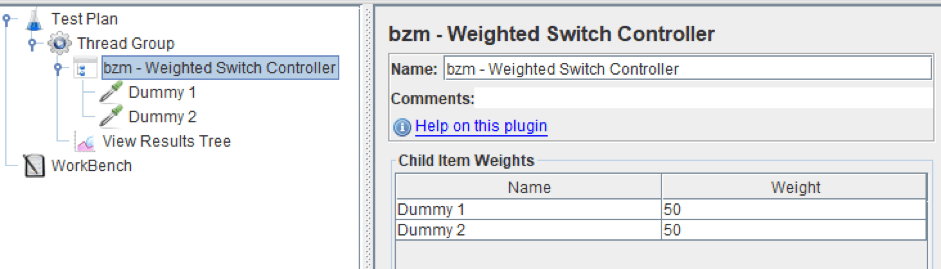6. Add a View Results Tree and run the script. The screenshot below shows the results for the following weights: 50/50, 75/25, 25/75, 90/10. As you can see, the results correspond to the proportions.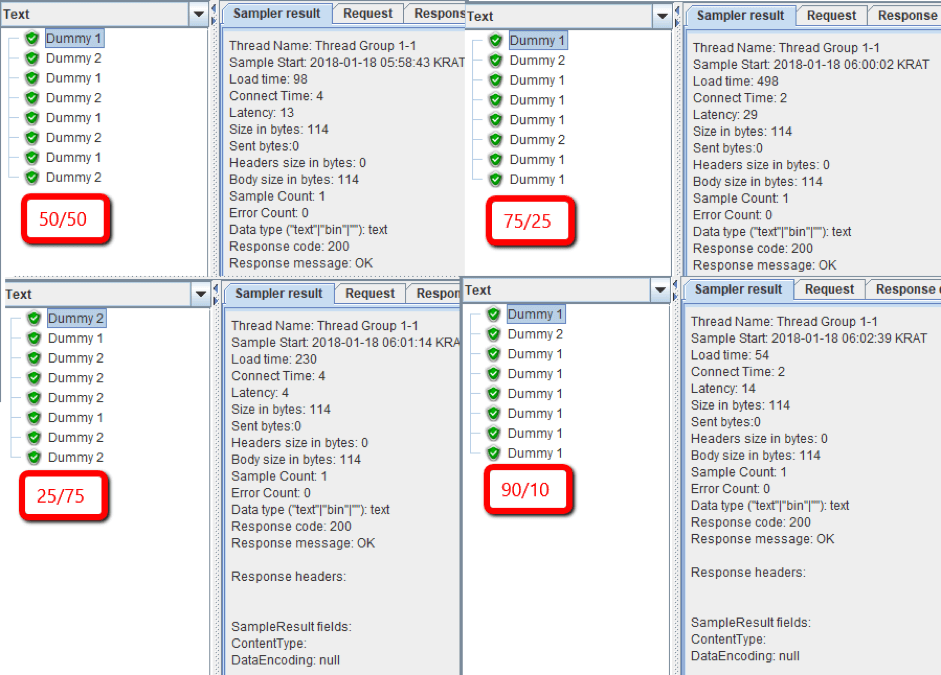## Using the Weighted Switch Controller with Multiple Elements in One Flow

If you have several elements that need to work in one flow, you need to logically combine them. This means that you must have an element that combines a group of samplers that should not be separated and should always be run together.

Usually a Simple Controller is used for this, but actually any controller can be used in this case.

2. Add a Weighted Switch Controller.

Right click on bzm - Weighted Switch Controller -> Add -> Logic Controller -> Simple Controller

4. Now, add 2 Dummy Samplers under the Switch Controller and a 3rd Dummy Sampler under the Weighted Switch Controller.

5. Configure the Weighted Switch Controller table and set the Simple Controller’s weight to 50 and Dummy 3’s weight to 50. As you can see in the screenshot below, there is no Dummy 1 or Dummy 2 in the Weighted Switch Controller table. So if the Simple Controller is run, then Dummy 1 and Dummy 2 will be executed sequentially.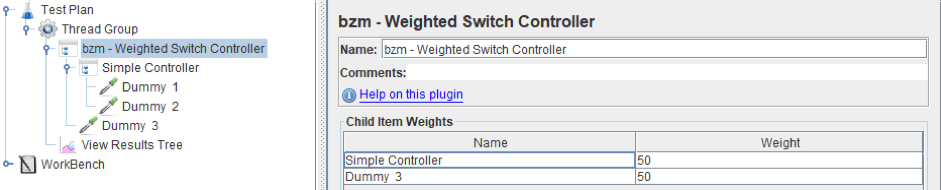6. Add a View Results Tree listener, run the script again and look at the results. As you can see, the controller works as stated. Dummy 1 + 2 appear 50% of the times, and Dummy 3 appears 50% of the times.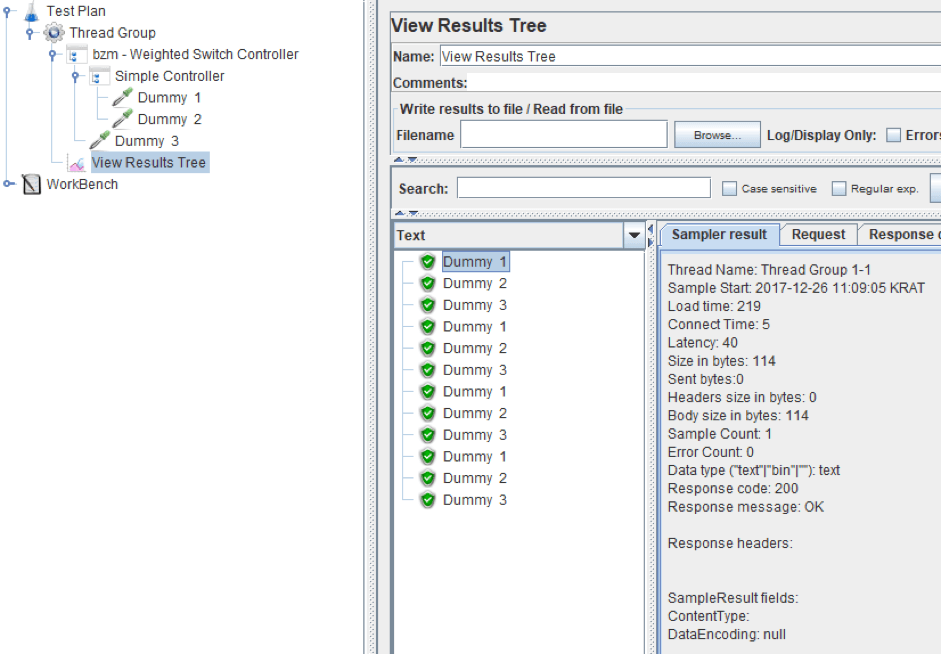### Setting the Number of Loops

In our example, we set the number of loops in the Thread Group to ensure the controller is executed several times. But you can also use other controllers for looping, like the Loop Controller and the While Controller.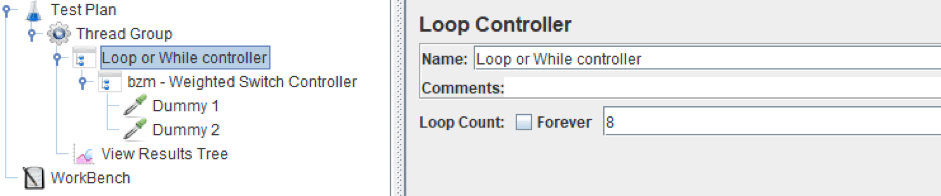The screenshot below shows the result of working through the loops of the Thread Group and For Controller. As you can see, the result is the same in both variants.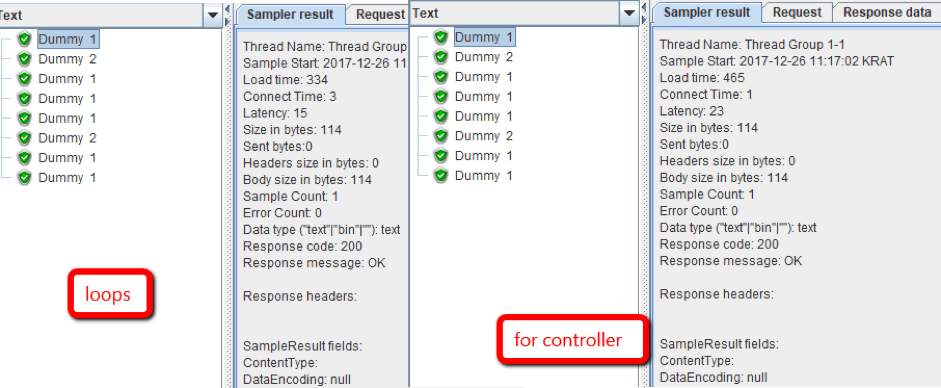## Weighted Switch Controller vs. JMeter Switch Controller

Before the Weighted Switch Controller, one of the ways to set the sampler execution frequency in load tests was the Switch Controller. But this solution had problems: you had to use JMeter functions and set up frequencies. (Learn Switch Controller solutions from this blog post “Running JMeter Samplers with Defined Percentage Probability”).

In the screenshot below you can see the same script fragment with the Switch Controller and the Weighted Switch Controller. It contains 4 elements, in our case 4 dummy samplers, each of which must be called with a certain frequency. You can see it’s much easier to use than the Switch Controller which requires creating functions. (Though you can also use functions in the Weighted Switch Controller).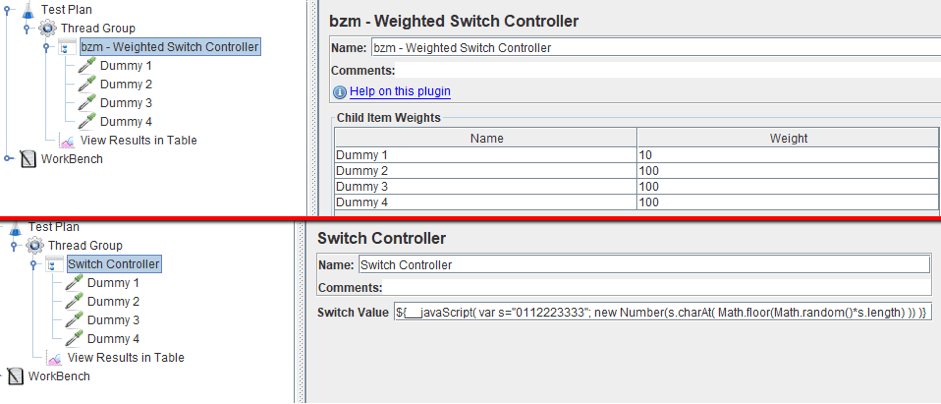### Important Notes

1. The controller does not select a value based on probability. No, it will not execute a random sampler out of two if each of them has a weight of 50. The gif below shows the result of 5 executions of the script with 75/25 configuration. As you can see, the order of the elements always remains the same.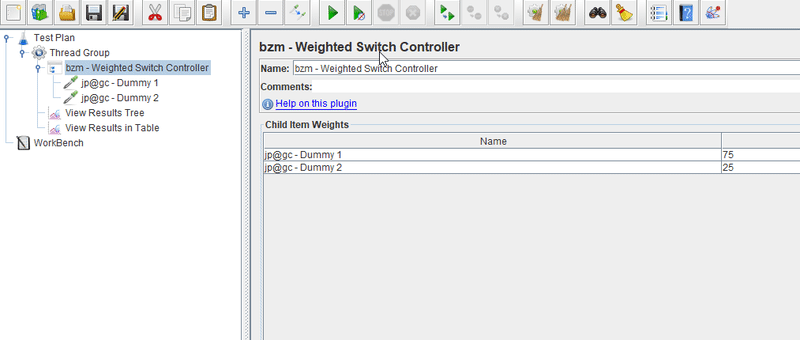2. In the case of adding a new child or renaming the existing child, the weight will be set a default value of 100. (In fact, the weight of the element will be zero, but 100 will be displayed. It will be 0 until you click on the field of the weighted switch controller. See point 4.). Be careful when you rename items and do not forget about this when you develop a script.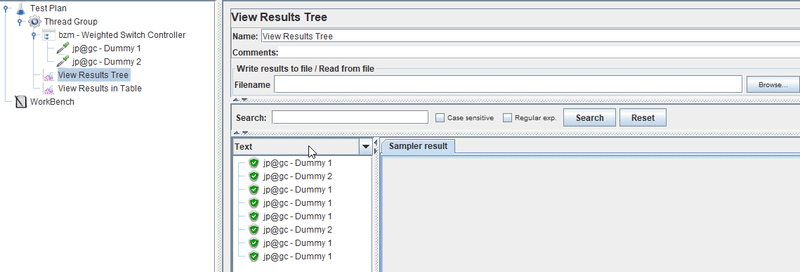3. If a child element is disabled, the relative weight of this element will not be counted in the test. It will not disappear from the list, but will not be used in calculations either and the weight of the other elements will be recalculated.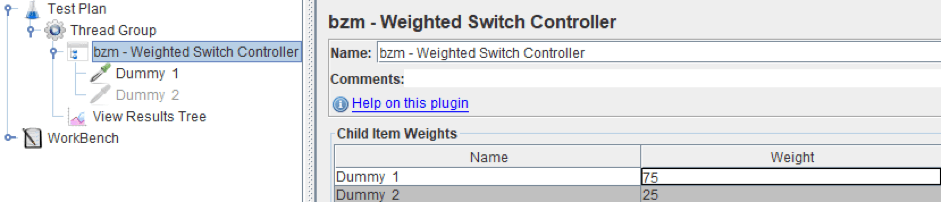4. A small annoying thing: when you are developing or debugging a script and changing the weights of the elements, do not forget to remove the focus from the cell in which the value was changed, otherwise it will not be applied while the script execution, but instead the old value for calculations will be used.

5. You do not specify the percentages, but the weight of the element. That is, you can use values that do not add up to 100, for example, 700/200 or 7/2 are the same, and you can use these values in the controller. But it is more convenient to use numbers that add up to 100 to draw an analogy with percentages.

6. A Weighted Switch Controller inside a Weighted Switch Controller will not work correctly, or more precisely the weights of the elements are not correctly calculated. The gif below shows a script with two controllers. The weight of each element is 50, 8 cycles are executed. Therefore, the result should be the following: Dummy 1 - 4 times, Dummy 2 - 2 times, Dummy 3 - 2 times, but it is different.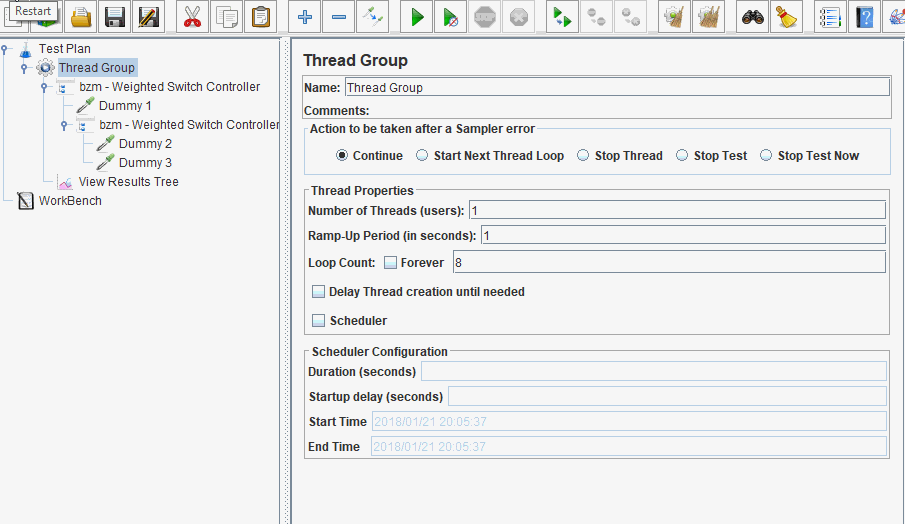### Conclusion

We have reviewed the Weighted Switch Controller plugin, which is designed to select an element with a certain frequency. It can be an excellent replacement for the Switch Controller in your tests. If we consider applying the controller in real life, then, for example, it is perfect for selecting the login path in the script or setting the frequency of certain requests to the database.